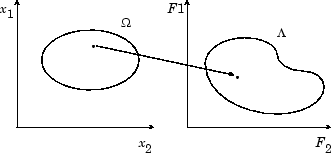## What Is Multiobjective Optimization?

You might need to formulate problems with more than one objective, since a single objective with several constraints may not adequately represent the problem being faced. If so, there is a vector of objectives,

 F(x) = [F1(x), F2(x),...,Fm(x)], (1)
that must be traded off in some way. The relative importance of these objectives is not generally known until the system's best capabilities are determined and tradeoffs between the objectives fully understood. As the number of objectives increases, tradeoffs are likely to become complex and less easily quantified. The designer must rely on his or her intuition and ability to express preferences throughout the optimization cycle. Thus, requirements for a multiobjective design strategy must enable a natural problem formulation to be expressed, and be able to solve the problem and enter preferences into a numerically tractable and realistic design problem.

Multiobjective optimization is concerned with the minimization of a vector of objectives F(x) that can be the subject of a number of constraints or bounds:

Note that because F(x) is a vector, if any of the components of F(x) are competing, there is no unique solution to this problem. Instead, the concept of noninferiority in Zadeh  (also called Pareto optimality in Censor  and Da Cunha and Polak ) must be used to characterize the objectives. A noninferior solution is one in which an improvement in one objective requires a degradation of another. To define this concept more precisely, consider a feasible region, Ω, in the parameter space. x is an element of the n-dimensional real numbers $x\in {ℝ}^{n}$ that satisfies all the constraints, that is,

`$\Omega =\left\{x\in {ℝ}^{n}\right\},$`

subject to

This allows definition of the corresponding feasible region for the objective function space Λ:

`$\Lambda =\left\{y\in {ℝ}^{m}:y=F\left(x\right),x\in \Omega \right\}.$`

The performance vector F(x) maps parameter space into objective function space, as represented in two dimensions in the figure Figure 9-1, Mapping from Parameter Space into Objective Function Space.

Figure 9-1, Mapping from Parameter Space into Objective Function SpaceA noninferior solution point can now be defined.

Definition: Point $x*\in \Omega$ is a noninferior solution if for some neighborhood of x* there does not exist a Δx such that $\left(x*+\Delta x\right)\in \Omega$ and

In the two-dimensional representation of the figure Figure 9-2, Set of Noninferior Solutions, the set of noninferior solutions lies on the curve between C and D. Points A and B represent specific noninferior points.

Figure 9-2, Set of Noninferior SolutionsA and B are clearly noninferior solution points because an improvement in one objective, F1, requires a degradation in the other objective, F2, that is, F1B < F1A, F2B > F2A.

Since any point in Ω that is an inferior point represents a point in which improvement can be attained in all the objectives, it is clear that such a point is of no value. Multiobjective optimization is, therefore, concerned with the generation and selection of noninferior solution points.

Noninferior solutions are also called Pareto optima. A general goal in multiobjective optimization is constructing the Pareto optima.

Watch now